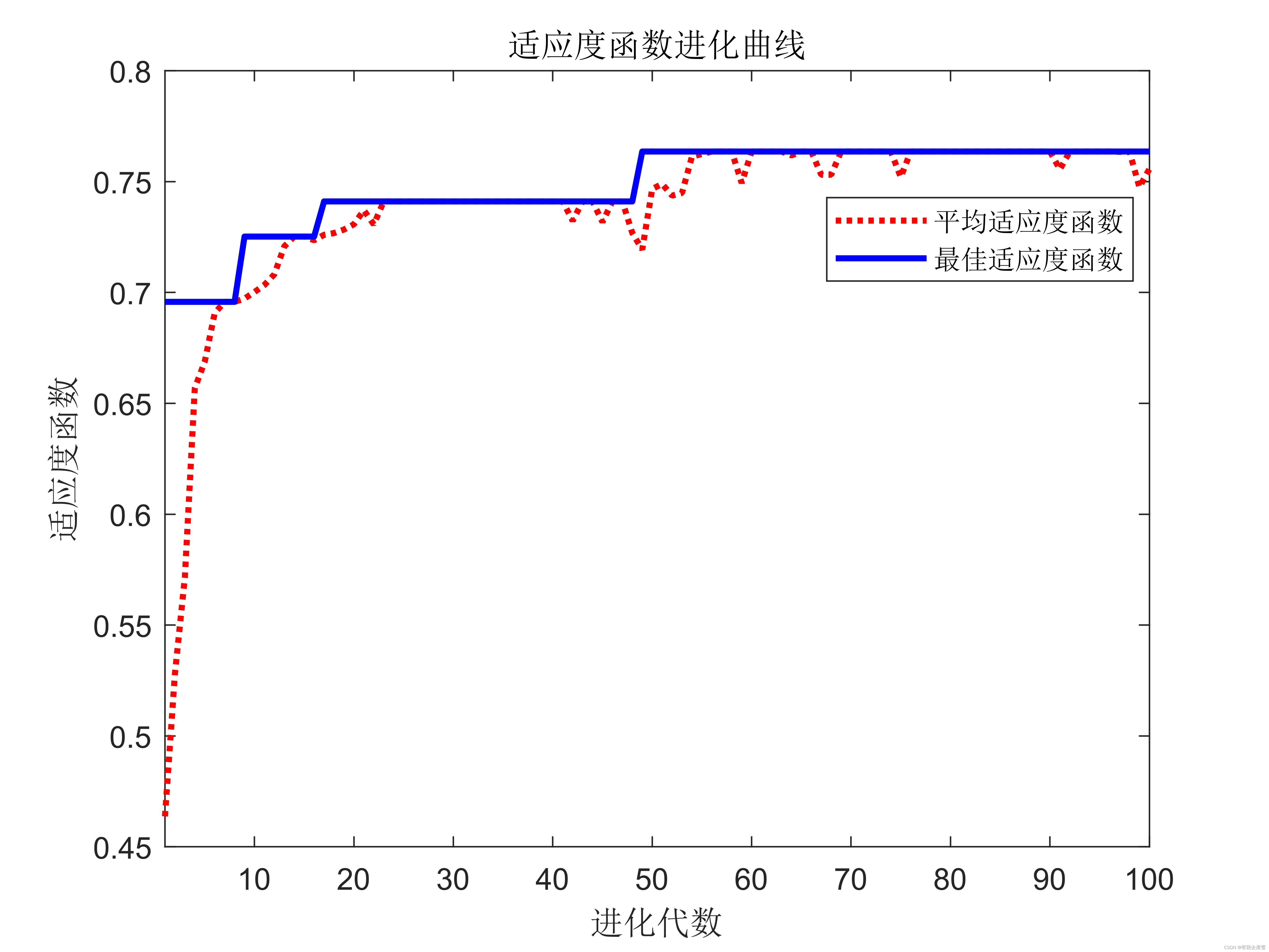## 使用遗传算法优化的BP神经网络实现自变量降维

news/2023/11/30 8:13:32

大家好，我是带我去滑雪！

在现实生活中，实际问题很难用线性模型进行描述。神经网络的出现大大降低了模型建立的难度和工作量。只需要将神经网络当作一个黑箱子，根据输入和输出数据，神经网络依据相关的学习规则，便可以建立相应的数学模型。但是，当数学模型的输入自变量（即影响因素）很多、输入自变量不是相互独立的时候，利用神经网络容易出现过拟合现象，从而导致所建立的模型精度低、建模时间长等问题。因此，在建立模型之前，有必要对输入自变量进行优化选择，将冗余的一些自变量去掉，选择最能反映输入与输出关系的自变量参与建模。常用的自变量压缩降维方法有多元回归与相关分析方法、类逐步回归法、主成分分析法、独立主成分分析法、主基地分析法、偏最小二乘法等等。本次利用遗传算法筛选出最具有代表的自变量，再利用BP神经网络进行预测。

（1）遗传算法

（2）问题描述

（3）模型建立

（1）清空环境变量，声明全局变量

（2）导入数据并归一化

（3）单BP神经网络创建、训练和仿真

（4） 遗传算法优化算法

（5）输入自变量优化解码子函数

（6） BP神经网络和阈值优化适应度子函数

（7） BP神经网络和阈值优化解码器子函数

（8）结果分析

### 一、问题提出与模型建立

#### （1）遗传算法

遗传算法(Genetic Algorithm)是一类借鉴生物界的进化规律(适者生存，优胜劣汰遗传机制)演化而来的随机化搜索方法。它是由美国的J.Holland教授1975年首先提出，其主要特点是直接对结构对象进行操作，不存在求导和函数连续性的限定;具有内在的隐并行性和更好的全局寻优能力;采用概率化的寻优方法，能自动获取和指导优化的搜索空间，自适应地调整搜索方向，不需要确定的规则。遗传算法的这些性质，已被人们广泛地应用于组合优化、机器学习、信号处理、自适应控制和人工生命等领域。

遗传算法的基本运算过程如下:

• a)初始化:设置进化代数计数器t=0，设置最大进化代数T，随机生成M个个体作为初始群体P(0)。
• b)个体评价:计算群体P(t)中各个个体的适应度。​编辑遗传算法
• c)选择运算:将选择算子作用于群体。选择的目的是把优化的个体直接遗传到下一代或通过配对交叉产生新的个体再遗传到下一代。选择操作是建立在群体中个体的适应度评估基础上的。
• d)交叉运算:将交叉算子作用于群体。遗传算法中起核心作用的就是交叉算子。
• e)变异运算:将变异算子作用于群体。即是对群体中的个体串的某些基因座上的基因值作变动。
• 群体P(t)经过选择、交叉、变异运算之后得到下一代群体P(t+1)。
• f)终止条件判断:若t=T,则以进化过程中所得到的具有最大适应度个体作为最优解输出，终止计算。

#### （2）问题描述

威斯康辛大学医学院经过多年的收集和整理，建立了一个乳腺肿瘤病灶组织的细胞核显微图像数据库。数据库包含了细胞核图像的10个量化特征（细胞核半径、质地、周长、面积、光滑性、紧密度、凹陷度、凹陷点数、对称度、断裂度）这些特征与肿瘤的性质有密切的关系。需要建立一个确定的模型来描述数据库中的各量化特征与肿瘤性质的关系，从而根据细胞核显微图像的量化特征诊断乳腺肿瘤是良性的还是恶性的。在建立模型的过程中，选择这10个特征的标准差、最坏值、平均值共30个数据。显然，这30个输入自变量之间存在一定的关系，并非互相独立的，因此，为了缩短建模时间、提高模型的精度，有必要将30个输入自变量中起主要影响因素的自变量筛选出来参与最终的建模。

#### （3）模型建立

首先利用遗传算法进行优化计算，需要将解空间映射到编码空间，每个编码对应问题的一个解（即染色体或个体）。这里，将编码长度设计为30，染色体的每一位对应一个输入自变量，每一位的基因取值只能是1和0两种情况，如果染色体某一位置是1，表示该位对应的输入自变量参与最终的建模；反之，则表示0对应的输入自变量不能作为最终的建模自变量。选取测试集数据均方误差的倒数作为遗传算法的适应度函数，这样经过不断的迭代进化，最终筛选出最具有代表性的输入自变量参与建模。

### 二、代码实现与结果分析

#### （1）清空环境变量，声明全局变量

``````clear all
clc
warning off
global P_train T_train P_test T_test  mint maxt S s1
S = 30;
s1 = 50;``````

#### （2）导入数据并归一化

为了保证结果的一般性，随机选取500组样本作为训练集，剩下的69组样本作为测试集。

``````load data.mat
a = randperm(569);
Train = data(a(1:500),:);
Test = data(a(501:end),:);
P_train = Train(:,3:end)';
T_train = Train(:,2)';
P_test = Test(:,3:end)';
T_test = Test(:,2)';
total_B = length(find(data(:,2) == 1));
total_M = length(find(data(:,2) == 2));
count_B = length(find(T_train == 1));
count_M = length(find(T_train == 2));
number_B = length(find(T_test == 1));
number_M = length(find(T_test == 2));
disp('实验条件为：');
disp(['病例总数：' num2str(569)...'  良性：' num2str(total_B)...'  恶性：' num2str(total_M)]);
disp(['训练集病例总数：' num2str(500)...'  良性：' num2str(count_B)...'  恶性：' num2str(count_M)]);
disp(['测试集病例总数：' num2str(69)...'  良性：' num2str(number_B)...'  恶性：' num2str(number_M)]);[P_train,minp,maxp,T_train,mint,maxt] = premnmx(P_train,T_train);
P_test = tramnmx(P_test,minp,maxp);``````

#### （3）单BP神经网络创建、训练和仿真

``````t = cputime;
net_bp = newff(minmax(P_train),[s1,1],{'tansig','purelin'},'trainlm');
% 设置训练参数
net_bp.trainParam.epochs = 1000;
net_bp.trainParam.show1 = 10;
net_bp.trainParam.goal = 0.1;
net_bp.trainParam.lr = 0.1;
net_bp.trainParam.showwindow = 0;
%% 训练单BP网络
net_bp = train(net_bp,P_train,T_train);
%% 仿真测试单BP网络
tn_bp_sim = sim(net_bp,P_test);
% 反归一化
T_bp_sim = postmnmx(tn_bp_sim,mint,maxt);
e = cputime - t;
T_bp_sim(T_bp_sim > 1.5) = 2;
T_bp_sim(T_bp_sim < 1.5) = 1;
result_bp = [T_bp_sim' T_test'];
%% 结果显示（单BP网络）
number_B_sim = length(find(T_bp_sim2 == 1 & T_test == 1));
number_M_sim = length(find(T_bp_sim == 2 &T_test == 2));
disp('(1)BP网络的测试结果为：');
disp(['良性乳腺肿瘤确诊：' num2str(number_B_sim)...'  误诊：' num2str(number_B - number_B_sim)...'  确诊率p1 = ' num2str(number_B_sim/number_B*100) '%']);
disp(['恶性乳腺肿瘤确诊：' num2str(number_M_sim)...'  误诊：' num2str(number_M - number_M_sim)...'  确诊率p2 = ' num2str(number_M_sim/number_M*100) '%']);
disp(['建模时间为：' num2str(e) 's'] );``````

#### （4） 遗传算法优化算法

利用遗传算法对自变量进行优化筛选时，染色体长度为30，种群大小设置为20，最大进化代数设置为100。

``````popu = 20;
bounds = ones(S,1)*[0,1];
% 产生初始种群
initPop = randi([0 1],popu,S);
% 计算初始种群适应度
initFit = zeros(popu,1);
for i = 1:size(initPop,1)initFit(i) = de_code(initPop(i,:));
end
initPop = [initPop initFit];
gen = 100;
% 优化计算
[X,EndPop,BPop,Trace] = ga(bounds,'fitness',[],initPop,[1e-6 1 0],'maxGenTerm',...gen,'normGeomSelect',0.09,'simpleXover',2,'boundaryMutation',[2 gen 3]);
[m,n] = find(X == 1);
disp(['优化筛选后的输入自变量编号为:' num2str(n)]);
% 绘制适应度函数进化曲线
figure
plot(Trace(:,1),Trace(:,3),'r:')
hold on
plot(Trace(:,1),Trace(:,2),'b')
xlabel('进化代数')
ylabel('适应度函数')
title('适应度函数进化曲线')
legend('平均适应度函数','最佳适应度函数')
xlim([1 gen])``````

#### （5）输入自变量优化解码子函数

``````function Val = de_code(x)
% 全局变量声明
global S P_train T_train P_test T_test mint maxt
global p t r s s1 s2
% 数据提取
x = x(:,1:S);
[m,n] = find(x == 1);
p_train = zeros(size(n,2),size(T_train,2));
p_test = zeros(size(n,2),size(T_test,2));
for i = 1:length(n)p_train(i,:) = P_train(n(i),:);p_test(i,:) = P_test(n(i),:);
end
t_train = T_train;
p = p_train;
t = t_train;
% 遗传算法优化BP网络权值和阈值
r = size(p,1);
s2 = size(t,1);
s = r*s1 + s1*s2 + s1 + s2;
aa = ones(s,1)*[-1,1];
popu = 20;  % 种群规模
initPpp = initializega(popu,aa,'gabpEval');  % 初始化种群
gen = 100;  % 遗传代数
% 调用GAOT工具箱，其中目标函数定义为gabpEval
x = ga(aa,'gabpEval',[],initPpp,[1e-6 1 0],'maxGenTerm',gen,...
'normGeomSelect',0.09,'arithXover',2,'nonUnifMutation',[2 gen 3]);
% 创建BP网络
net = newff(minmax(p_train),[s1,1],{'tansig','purelin'},'trainlm');
% 将优化得到的权值和阈值赋值给BP网络
net.IW{1,1} = W1;
net.LW{2,1} = W2;
net.b{1} = B1;
net.b{2} = B2;
% 设置训练参数
net.trainParam.epochs = 1000;
net.trainParam.show = 10;
net.trainParam.goal = 0.1;
net.trainParam.lr = 0.1;
net.trainParam.showwindow = 0;
% 训练网络
net = train(net,p_train,t_train);
% 仿真测试
tn_sim = sim(net,p_test);
% 反归一化
t_sim = postmnmx(tn_sim,mint,maxt);
% 计算均方误差
SE = sse(t_sim - T_test);
% 计算适应度函数值
Val = 1/SE;
end``````

#### （6） BP神经网络和阈值优化适应度子函数

``````function[sol,val] = gabpEval(sol,options)
global s
for i = 1:s
x(i) = sol(i);
end;

#### （7） BP神经网络和阈值优化解码器子函数

``````function[W1,B1,W2,B2,val] = gadecod(x)
global p t r s1 s2
W1 = zeros(s1,r);
W2 = zeros(s2,s1);
B1 = zeros(s1,1);
B2 = zeros(s2,1);
% 前r*s1个编码为W1
for i = 1:s1for k = 1:rW1(i,k) = x(r*(i-1)+k);end
end
% 接着的s1*s2个编码(即第r*s1个后的编码)为W2
for i = 1:s2for k = 1:s1W2(i,k) = x(s1*(i-1)+k+r*s1);end
end
% 接着的s1个编码(即第r*s1+s1*s2个后的编码)为B1
for i = 1:s1B1(i,1) = x((r*s1+s1*s2)+i);
end
% 接着的s2个编码(即第r*s1+s1*s2+s1个后的编码)为B2
for i = 1:s2B2(i,1) = x((r*s1+s1*s2+s1)+i);
end
% 计算S1与S2层的输出
A1 = tansig(W1*p,B1);
A2 = purelin(W2*A1,B2);
% 计算误差平方和
SE = sumsqr(t - A2);
% 遗传算法的适应值
val = 1/SE;``````

#### （8）结果分析

(2)优化BP网络的测试结果为：

经过遗传算法优化计算后，筛选出的一组输入自变量编号为：1   2   3   4   6   9  10  12  13  14  15  19  20  22  25  27  28  29  30，筛选的18个自变量。种群适应度函数的进化曲线为：经过遗传算法优化优化筛选后，参与建模的输入自变量大大减少，并且建模时间大大减少。

点赞+关注,下次不迷路！

### Unreal引擎自带的有用的工具函数，持续更新中

Unreal引擎自带的有用的工具函数&#xff0c;持续更新中 1、计算三角形法线、面积等的函数 Epic Games\UE_x.x\Engine\Source\Runtime\GeometryCore\Public\VectorUtil.h 2、计算FMeshDescription的切线、法线和简单UV等的函数 Epic Games\UE_x.x\Engine\Source\Runtime\Static…

### [java进阶]——泛型类、泛型方法、泛型接口、泛型的通配符

&#x1f308;键盘敲烂&#xff0c;年薪30万&#x1f308; 目录 泛型的基础知识&#xff1a; ♥A 泛型的好处&#xff1a; ♠A 泛型擦除&#xff1a; ♣A 泛型的小细节&#xff1a; 泛型的使用&#xff1a; ①泛型类&#xff1a; ②⭐泛型接口&#xff1a; ③泛型方法&…